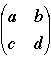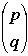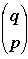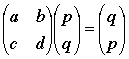SEARCH HOMEMath Central Quandaries & QueriesQuestion from tousif, a student: Find the 2 x 2 matrix which represents a reflection in the line y = x? Please help....Hi Tousif,

Suppose the matrix isandis a point in the plane. Under a reflection in the line y = x the pointis transformed to. ThusExpand this matrix equation to yield two linear equations. These equations are valid for all choices of p and q. What happens when p = 0 and q = 1? What does this tell you abut the transformation matrix?

PennyMath Central is supported by the University of Regina and The Pacific Institute for the Mathematical Sciences.